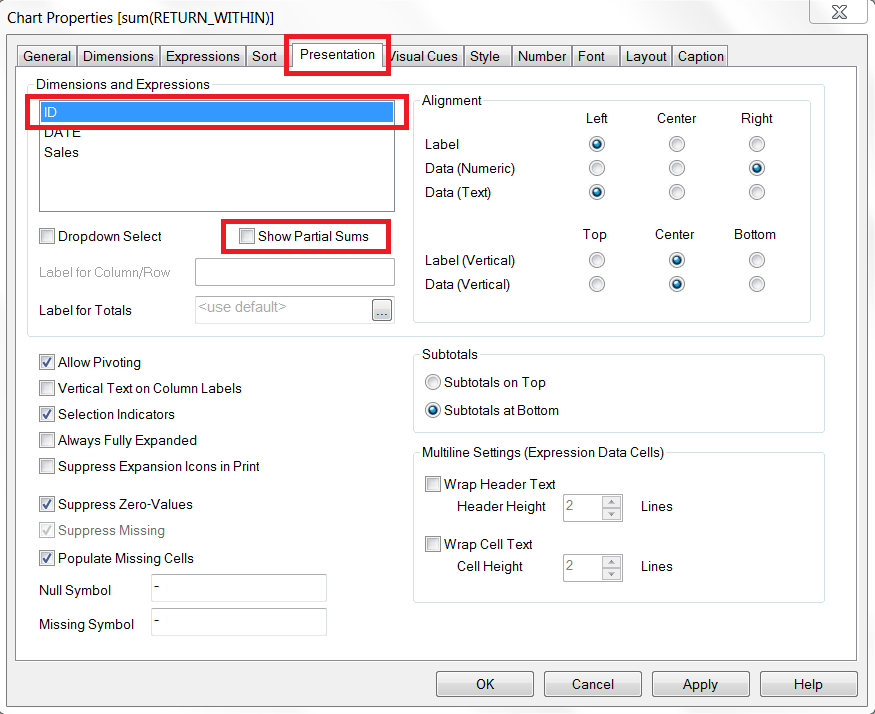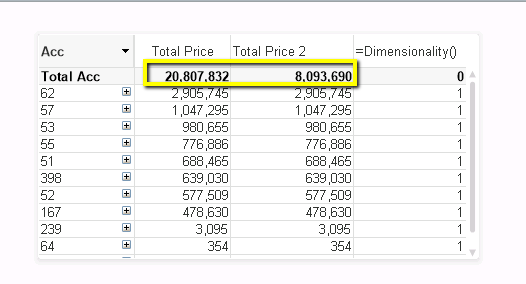# QlikView App Development

Discussion Board for collaboration related to QlikView App Development.

Highlighted
Contributor III

## Pivot with 3 dimensions Avg(), Sum() Dimensionality()

Hi,

I need to create a pivot for data which have the same price for a part  each line of a file.

The pivot dimensions should be Acc Family, Family, part.

The measure to be calculated  is total price.

I need to show sub totals for each dim.

The challenge is to manipulate between Avg and Sum.

Which is the best way to achieve this?

By doing some manipulation in script?

I got a bit lost with Dimensioanlity & aggr..

Attached is some sample data and the model.

I would appreciate some guidelines about the way to deal with such a case.

Thanks!Tags (2)
1 Solution

Accepted SolutionsPartner

## Re: Pivot with 3 dimensions Avg(), Sum() Dimensionality()

Not sure if you need to deal with dimensionality here at all - this formula:

Sum(Aggr(Avg([Total Price]), [Acc Family], Family, Part))

will show averages at the Part level, and sum them up on Family and Acc Family levels.

On the other hand, this gives exact same numbers as your old formula, so I'm not sure if I understood your requirements correctlyIf you have 2 different Total Prices for the same Part, do you actually want to have them averaged, or summed (distinct values only, not every repeated one)?

5 RepliesMVP

## Re: Pivot with redundant value

If you create a pivot table, you can select to see sub-totals for any of your dimensions on the presentation tab:You need to select each dimension and check the "Show Partial Sums" box for each one you want to see the subtotal for.

Contributor III

## Re: Pivot with 3 dimensions Avg(), Sum() Dimensionality()

Hi Nicole,

Thank you for your tip.

But in this case that solution will generate wrong results, since the value to be summed repeats itself in the data. Another words, the expression has to be average and not sum, and I have problems with getting the right dimensionality expressions..

I attached a model to demonstrate it.

Thanks!Partner

## Re: Pivot with 3 dimensions Avg(), Sum() Dimensionality()

Not sure if you need to deal with dimensionality here at all - this formula:

Sum(Aggr(Avg([Total Price]), [Acc Family], Family, Part))

will show averages at the Part level, and sum them up on Family and Acc Family levels.

On the other hand, this gives exact same numbers as your old formula, so I'm not sure if I understood your requirements correctlyIf you have 2 different Total Prices for the same Part, do you actually want to have them averaged, or summed (distinct values only, not every repeated one)?

Contributor III

## Re: Pivot with 3 dimensions Avg(), Sum() Dimensionality()

Hi Jakub,

I added your expression to the model (Total Price 2), and you can see that it doesn't show the exact same numbers.

Looks like your expression is the correct one 🙂

The data structure is that a part a few costs line, and each has the part price (which is identical). That's why i needed the average,

but also show a correct total for the 3 dimensions..

Thank you!Contributor III

## Re: Pivot with 3 dimensions Avg(), Sum() Dimensionality()

Sorry..

You were right.. i had the same numbers... forgot that i fooled around with the expression..

But looks like expression is what I need..

Thanks Again!